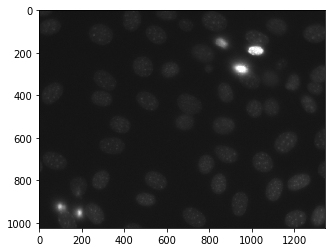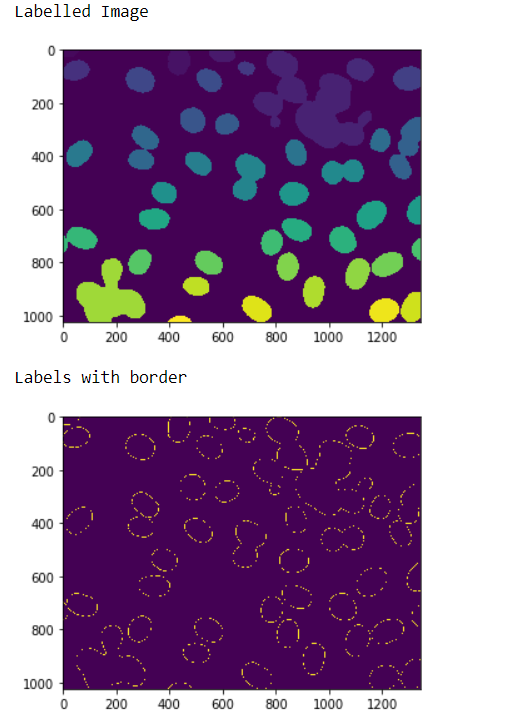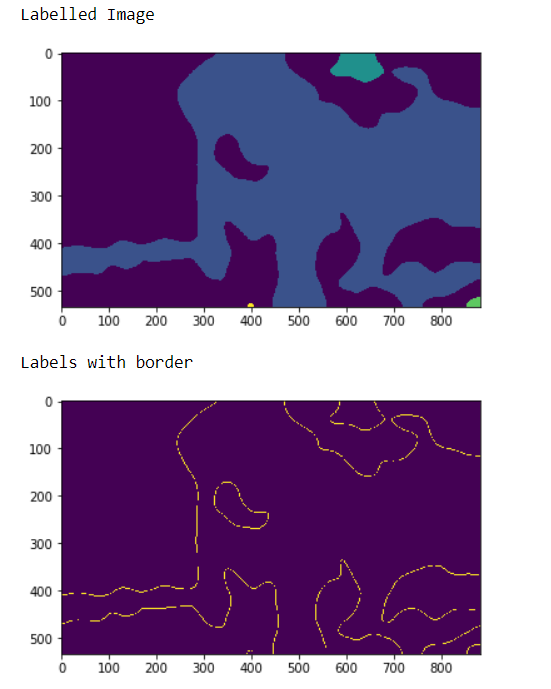# Mahotas – Getting Border of labels

In this article we will see how we can get the borders of the labels in the labelled image in mahotas. For this we are going to use the fluorescent microscopy image from a nuclear segmentation benchmark. We can get the image with the help of command given below

`mhotas.demos.nuclear_image()`

Below is the nuclear_imageIn order to do this we will use `mahotas.labeled.borders` method

Syntax : mahotas.labeled.borders(labeled_image)

Argument : It takes numpy.ndarray object as argument i.e labelled image

Return : It returns numpy.ndarray object i.e labeled image with only labels border

Note : The input of the this should should be the filtered image object which is labeled
In order to filter the image we will take the image object which is numpy.ndarray and filter it with the help of indexing, below is the command to do this

`iamge = image[:, :, 0]`

Example 1 :

 `# importing required libraries ` `import` `mahotas ` `import` `numpy as np ` `from` `pylab ``import` `imshow, show ` `import` `os ` ` `  `# loading nuclear image ` `f ``=` `mahotas.demos.load(``'nuclear'``) ` ` `  `# setting filter to the image ` `f ``=` `f[:, :, ``0``] ` ` `  `# setting gaussian filter ` `f ``=` `mahotas.gaussian_filter(f, ``4``) ` ` `  `# setting threshold value ` `f ``=` `(f> f.mean()) ` ` `  `# creating a labeled image ` `labeled, n_nucleus ``=` `mahotas.label(f) ` ` `  ` `  `# showing the labeleed image ` `print``(``"Labelled Image"``) ` `imshow(labeled) ` `show() ` ` `  `# getting border of the labeled image ` `relabeled ``=` `mahotas.labeled.borders(labeled) ` ` `  `# showing the image ` `print``(``"Labels with border"``) ` `imshow(relabeled) ` `show() `

Output :Example 2 :

 `# importing required libraries ` `import` `numpy as np ` `import` `mahotas ` `from` `pylab ``import` `imshow, show ` `  `  `# loading iamge ` `img ``=` `mahotas.imread(``'dog_image.png'``) ` `    `  `# filtering the imagwe ` `img ``=` `img[:, :, ``0``] ` `     `  `# setting gaussian filter ` `gaussian ``=` `mahotas.gaussian_filter(img, ``15``) ` `  `  `# setting threshold value ` `gaussian ``=` `(gaussian > gaussian.mean()) ` `  `  `# creating a labeled image ` `labeled, n_nucleus ``=` `mahotas.label(gaussian) ` `   `  `print``(``"Labelled Image"``) ` `# showing the gaussian filter ` `imshow(labeled) ` `show() ` `  `  `# getting border of the labeled image ` `relabeled ``=` `mahotas.labeled.borders(labeled) ` ` `  `# showing the image ` `print``(``"Labels with border"``) ` `imshow(relabeled) ` `show() `

Output :My Personal Notes arrow_drop_upCheck out this Author's contributed articles.

If you like GeeksforGeeks and would like to contribute, you can also write an article using contribute.geeksforgeeks.org or mail your article to contribute@geeksforgeeks.org. See your article appearing on the GeeksforGeeks main page and help other Geeks.

Please Improve this article if you find anything incorrect by clicking on the "Improve Article" button below.

Article Tags :

Be the First to upvote.

Please write to us at contribute@geeksforgeeks.org to report any issue with the above content.